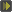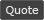WoWInterface - View Single Post - BloodyScreen, Renovation for MoP
 View Single Post
06-12-13, 07:13 AM   #38
Resike
A Pyroguard Emberseer
Join Date: Mar 2010
Posts: 1,289
 Originally Posted by humfrasWouldn't it be alot easier to have multiple spawn areas? Get random spawn area from all available => Get random point in that area => blood!
Well this is still in alpha, but i think storing multiple draw areas just a waste of code. The random generator works fine, so actually the bloods gets popped totally randomly.
My current method works like this:

Code:
```        TextureBlood[i]:SetTexture(AddonPath.."Textures\\"..BloodyMaskVars.TextureBlood.Type.."\\Blood"..((i % BM_NumberOfTextureFiles) + 1).."_1")
TextureBlood[i]:ClearAllPoints()
local Width = (UIParent:GetWidth() * BloodyMaskVars.TextureBloodFrame.Width) - (TextureBlood[i]:GetWidth() * math.sqrt(2))
local Height = (UIParent:GetHeight() * BloodyMaskVars.TextureBloodFrame.Height) - (TextureBlood[i]:GetHeight() * math.sqrt(2))
local x = math.random(Width) - (Width / 2)
local y = math.random(Height) - (Height / 2)
local PreventWidth = UIParent:GetWidth() * 0.1
local PreventHeight = UIParent:GetHeight() * (UIParent:GetWidth() / UIParent:GetHeight()) / 10
if x > - PreventWidth and x <= 0 and y > - PreventHeight and y <= 0 then
x = (PreventWidth + math.random(25)) * - 1
y = (PreventHeight + math.random(25)) * - 1
elseif x > - PreventWidth and x <= 0 and y < PreventHeight and y > 0 then
x = (PreventWidth + math.random(25)) * - 1
y = PreventHeight + math.random(25)
elseif x < PreventWidth and x > 0 and y > - PreventHeight and y <= 0 then
x = PreventWidth + math.random(25)
y = (PreventHeight + math.random(25)) * - 1

elseif x < PreventWidth and x > 0 and y < PreventHeight and y > 0 then
x = PreventWidth + math.random(25)
y = PreventHeight + math.random(25)
end
TextureBlood[i]:SetPoint("CENTER", TextureBloodFrame, "CENTER", x, y)```

Last edited by Resike : 06-12-13 at 07:15 AM.